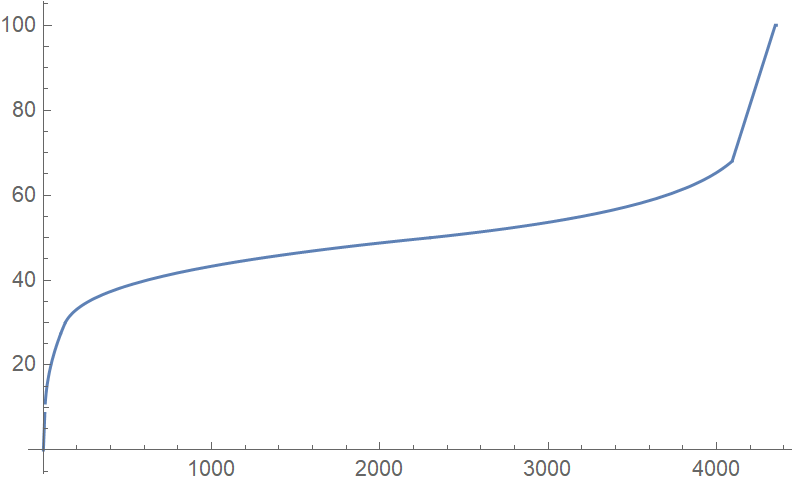# #679. 无标号图编码

### 评测方式

• 评测程序会运行你的程序，输入两行，第一行是单个字母 A，第二行是一个长度为 $n$ 的比特串。你需要输出一张 $M$ 个顶点的无向图，具体格式见下文。
• 评测程序会打乱这张无向图的点标号和边标号。
• 评测程序会重新运行你的程序，输入若干行，第一行是单个字母 B，第二行及以后表示一张 $M$ 个顶点的无向图。你的程序需要输出一个长度为 $n$ 的比特串，且输出串需要和这一轮最开始输入的串相同。

### 范例代码与执行过程

#include <bits/stdc++.h>
using namespace std;
int main() {
int n = 1;
string s;
cin >> s;
if (s == "N") {
cout << n << endl;
} else if (s == "A") {
string z;
cin >> z;
if (z == "0") {
cout << 0 << endl;
} else {
cout << 1 << endl;
cout << 1 << ' ' << 2 << endl;
}
} else if (s == "B") {
int e;
cin >> e;
if (e == 0) {
cout << "0" << endl;
} else if (e == 1) {
cout << "1" << endl;
}
}
}

A
0

A
1

0

1
1 2

B
0

B
1
11 45

### 给分方式

$$\mathrm{score} = \left\lfloor\frac{f(u)}{f(0)}g(x)\right\rfloor,$$

$$f(u)=\max{\left(\frac{1}{1+u}-\frac{1}{51},0\right)}$$

$$g(x)= \begin{cases} x,&0 < x\le10\\ 2\sqrt{x-9}+8,&10 < x\le130\\ 2\sqrt{x-103}+24,&130 < x\le2300\\ -6\log_2\left(4348-x\right)+116,&2300 < x\le4092\\ \frac{x}{8}-\frac{887}{2},&4092 < x\le4348\\ 100,&4348 < x\le4355\\ x-4255,&x > 4355 \end{cases}$$